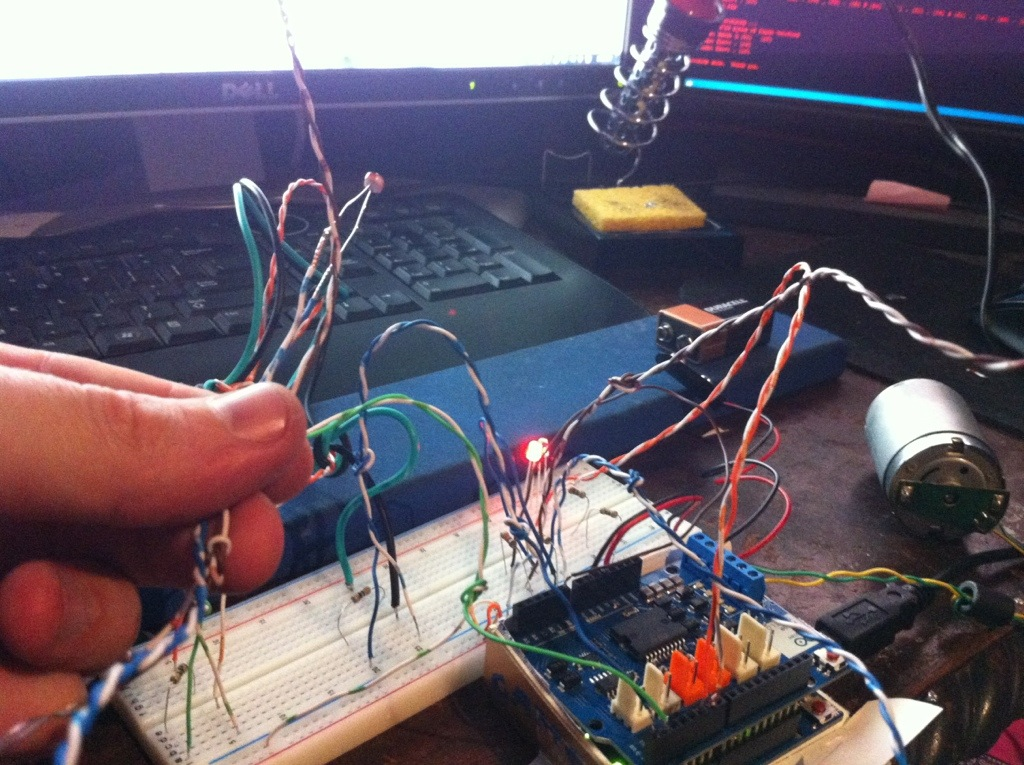# Arduino based Solar Panel Tracker

Arduino based Solar Tracker for Solar Panels

Works via two photoresistors.Here is the Arduino Sketch code I wrote for the tracker:

``` int lightPin = A0; //define a pin for Photo resistor int ledPin=6; //define a pin for LED int val= 1; int lightPin1 = A1; //define a pin for Photo resistor int ledPin5=5; //define a pin for LED int val1= 1; int setPoint19 = 20; // Trigger value int setPoint25 = 25; // Trigger value int setPoint29 = 29; // Trigger value int setPoint35 = 35; // Trigger value void setup() { Serial.begin(9600); //Begin serial communcation pinMode( ledPin, OUTPUT ); pinMode( ledPin5, OUTPUT ); } void loop() { val = (analogRead(lightPin)*1); if (val < setPoint35) { analogWrite(ledPin, LOW); // It has got dark, turn the LED on. } else { analogWrite(ledPin, 255); // It is light again, turn the LED off. } Serial.print("val = "); Serial.print(val ) ; //Write the value of the photoresistor to the serial monitor. Serial.print(" lightPin = "); Serial.print(analogRead(lightPin)); Serial.print(" lightPin /4-1= "); Serial.println(analogRead(lightPin)/4-1); //Write the value of the photoresistor to the serial monitor. //analogWrite(ledPin, val); //send the value to the ledPin. Depending on value of resistor //you have to divide the value. for example, //with a 10k resistor divide the value by 2, for 100k resistor divide by 4. //Serial.println(analogRead(lightPin)/3); //Write the value of the photoresistor to the serial monitor. delay(10); //short delay for faster response to light. val1 = (analogRead(lightPin1)*1); if (val1 < setPoint35) { analogWrite(ledPin5, LOW); // It has got dark, turn the LED on. } else { analogWrite(ledPin5, 255); // It is light again, turn the LED off. } Serial.print("val1= "); Serial.print(val1 ) ; //Write the value of the photoresistor to the serial monitor. Serial.print(" lightPin1= "); Serial.print(analogRead(lightPin1)); Serial.print(" lightPin1/4-1= "); Serial.println(analogRead(lightPin1)/4-1); //Write the value of the photoresistor to the serial monitor. //analogWrite(ledPin, val); //send the value to the ledPin. Depending on value of resistor //you have to divide the value. for example, //with a 10k resistor divide the value by 2, for 100k resistor divide by 4. //Serial.println(analogRead(lightPin)/3); //Write the value of the photoresistor to the serial monitor. delay(10); //short delay for faster response to light. ```

```} ```

Arduino Solar Tracker

Here is the Arduino sketch code I wrote that will move a 2-wire DC motor forward and reverse depending on which of 2 buttons is pressed:
``` #include ```

``` /* * Switch and LED test program */ int ledPin = 5; // LED is connected to pin 12 int switchPin = 0; // switch is connected to pin 2 int val; // variable for reading the pin status int ledPin6 = 6; // LED is connected to pin 12 int switchPin1 = 1; // switch is connected to pin 2 int val1; // variable for reading the pin status void setup() { pinMode(ledPin, OUTPUT); // Set the LED pin as output pinMode(switchPin, INPUT); // Set the switch pin as input pinMode(ledPin6, OUTPUT); // Set the LED pin as output pinMode(switchPin1, INPUT); // Set the switch pin as input //Setup Channel A pinMode(12, OUTPUT); //Initiates Motor Channel A pin pinMode(9, OUTPUT); //Initiates Brake Channel A pin } void loop(){ delay(10); val = digitalRead(switchPin); // read input value and store it in val if (val == LOW) { // check if the button is pressed digitalWrite(ledPin, HIGH); // turn LED on //Serial.begin(9600); // set up Serial library at 9600 bps //Serial.print("sw0 on: ");Serial.println(digitalRead(switchPin)); //forward @ full speed digitalWrite(12, HIGH); //Establishes forward direction of Channel A digitalWrite(9, LOW); //Disengage the Brake for Channel A analogWrite(3, 255); //Spins the motor on Channel A at full speed delay(3000); //delay(10); } if (val == HIGH) { // check if the button is not pressed digitalWrite(ledPin, LOW); // turn LED off delay(10); digitalWrite(9, HIGH); //Eengage the Brake for Channel A //delay(1000); } delay(10); val = digitalRead(switchPin1); // read input value and store it in val if (val == LOW) { // check if the button is pressed digitalWrite(ledPin6, HIGH); // turn LED on //Serial.print("on: ");Serial.println(digitalRead(switchPin1)); //backward @ half speed digitalWrite(12, LOW); //Establishes backward direction of Channel A digitalWrite(9, LOW); //Disengage the Brake for Channel A analogWrite(3, 255); //Spins the motor on Channel A at half speed delay(3000); delay(10); } if (val == HIGH) { // check if the button is not pressed digitalWrite(ledPin6, LOW); // turn LED off //Serial.print("1=off: ");Serial.println(digitalRead(switchPin1)); digitalWrite(9, HIGH); //Eengage the Brake for Channel A delay(1000); delay(10); } ```

```} ```

## 2 Replies to “Arduino based Solar Panel Tracker”

1.Gil says:

Hello. Good work. Do you have the schematic for this project?

1.admin says:

no but it is very basic. google ‘arduino photocell’